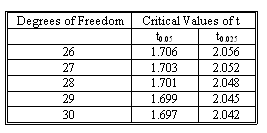### CFA Practice Question

There are 208 practice questions for this study session.

### CFA Practice Question

In a multiple regression model based on 30 observations and 2 independent variables, you have the estimate of b1 equal to 1.25. The standard error of this estimate is .09. Find the 95% confidence interval for the parameter b1.